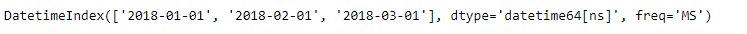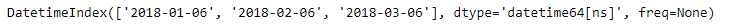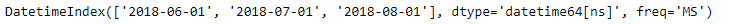Related Articles

# Python | Pandas Index.shift()

• Last Updated : 18 Dec, 2018

Python is a great language for doing data analysis, primarily because of the fantastic ecosystem of data-centric python packages. Pandas is one of those packages and makes importing and analyzing data much easier.

Pandas` Index.shift()` function shift index by desired number of time frequency increments. This method is for shifting the values of datetime-like indexes by a specified time increment a given number of times. This method is only implemented for datetime-like index classes, i.e., DatetimeIndex, PeriodIndex and TimedeltaIndex.

Syntax: Index.shift(periods=1, freq=None)

Parameters :
periods : Number of periods (or increments) to shift by, can be positive or negative.
freq : [pandas.DateOffset, pandas.Timedelta or string, optional] Frequency increment to shift by. If None, the index is shifted by its own freq attribute. Offset aliases are valid strings, e.g., ‘D’, ‘W’, ‘M’ etc

Returns : shifted index

Example #1: Use `Index.shift()` function to shift a time-series data by certain duration.

 `# importing pandas as pd``import` `pandas as pd`` ` `# Creating the index ``idx ``=` `pd.date_range(``'1 / 1/2018'``, periods ``=` `3``, freq ``=``'MS'``)`` ` `# Print the index``idx`

Output :Now we would shift the index by 5 Days.

 `# shifting the index by 5 days``idx.shift(``5``, freq ``=``'D'``)`

Output :As we can see in the output, the dates have been shifted forward by 5 Days.

Example #2: Use `Index.shift()` function shift the date-time based index.

 `# importing pandas as pd``import` `pandas as pd`` ` `# Creating the index ``idx ``=` `pd.date_range(``'1 / 1/2018'``, periods ``=` `3``, freq ``=``'MS'``)`` ` `# Print the index``idx`

Output :Now we would shift the index by 5 Months.

 `# shifting the index by 5 Months``idx.shift(``5``, freq ``=``'MS'``)`

Output :As we can see in the output, the date has been shifted forward by 5 months.

Attention geek! Strengthen your foundations with the Python Programming Foundation Course and learn the basics.

To begin with, your interview preparations Enhance your Data Structures concepts with the Python DS Course. And to begin with your Machine Learning Journey, join the Machine Learning – Basic Level Course

My Personal Notes arrow_drop_up